Practice the AP 10th Class Physical Science Bits with Answers Chapter 8 Chemical Bonding on a regular basis so that you can attempt exams with utmost confidence.

## AP State Syllabus 10th Class Physical Science Bits 8th Lesson Chemical Bonding with Answers

CONCEPT -1: Octet Rule & Lewis Dot Structures

Question 1.
By the late 19th century and early twentieth century the forces known by scientists are ……………..
A) Gravitational
B) Magnetic
C) Electrostatic
D) All of these
D) All of these

Question 2.
Atoms tends to combine when
A) Attraction is more than repulsion
B) Attraction is less than repulsion
C) Attraction is equal to repulsion
D) None of these
A) Attraction is more than repulsion

Question 3.
Valance shell means ……………..
A) inner shell
B) middle shell
C) outermost shell
D) both A and B
C) outermost shellQuestion 4.
Electrons in …………….. shell are responsible for the formation of bonds between atoms.
A) inner
B) valance
C) outermost
D) both B and C
D) both B and C

Question 5.
Noble gases belong to …………….. group
A) VIIIA
B) 18th
C) 0
D) All of these
D) All of these

Question 6.
…………….. exhibits octet configuration.
A) Transition elements
B) Inner transition elements
C) Representative elements
D) Noble gases
D) Noble gases

Question 7.
The valance electrons in the atom of an element is depicted in short form by …………….. symbol or …………….. dot structure
A) Newton, electron
B) Lewis, electron
C) Bohr, electron
D) Lewis, proton
B) Lewis, electron

Question 8.
The Lewis dot structure of Argon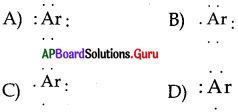A

Question 9.
Match the following: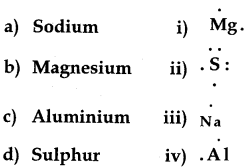A) a → i, b → ii, c → iii, d → iv
B) a → iv, b → iii, c → ii, d → i
C) a → iii, b → i, c → iv, d → ii
D) a → iv, b → i, c → ii, d → iii
C) a → iii, b → i, c → iv, d → ii

Question 10.
Match the following:
Group — Valency
a) I group — i) +2
b) II group — ii) -1
c) VI group — iii) + 1
d) VII group — iv) -2
A) a → i, b → ii, c → iii, d → iv
B) a → iii, b → i, c → iv, d → ii
C) a → iv, b → iii, c → ii, d → i
D) a → iii, b → iv, c → ii, d → i
B) a → iii, b → i, c → iv, d → ii

Question 11.
Statement I: Eight electrons in the outermost shell give stability to the ion or atom.
Statement II: Kernel is the nucleus and all other electrons in the atom except the outer most shell electrons.
A) Both statements are true
B) Statement I is true and statement II is false
C) Statement I is false and statement II is true
D) Both are false
A) Both statements are true

Question 12.
All inert gases except He have …………….. configuration
A) doublet
B) triplet
C) octet
D) helptet
C) octetQuestion 13.
The inert gas which does not have octet configuration ……………..
A) Argon
B) Helium
C) Neon
D) Krypton
B) Helium

Question 14.
The force of attraction between any two atoms or a group of atoms that results in a stable entity is called …………………
A) Physical bond
B) Chemical bond
C) Native bond
D) None of these
B) Chemical bond

Question 15.
Chemical bond among these is ……………..
A) Ionic bond
B) Covalent bond
C) Polar covalent bond
D) All of these
D) All of these

Question 16.
Ionic bond was proposed by ……………..
A) Kossel
B) G.N. Lewis
C) Linus Pauling
D) Sidgwick
A) Kossel

Question 17.
Transfer of electrons from one atom to other atom leads to…………….. bond.
A) Ionic bond
B) Covalent bond
C) Polar covalent bond
D) Coordinate covalent bond
A) Ionic bond

Question 18.
Metals generally form ……………..
A) cation
B) anion
C) positive ion
D) both A and C
D) both A and C

Question 19.
Non metals generally form ……………..
A) cation
B) anion
C) negative ion
D) both B and C
D) both B and C

Question 20.
The valency of I, II, III groups respectively are ……………..
A) 1, 2, 3
B) 3, 2,1
C) 4, 5, 6
D) 0,1, 2
A) 1, 2, 3Question 21.
The valency of V, VI, VII groups respectively are………………
A) 1, 2, 3
B) 3, 2,1
C) 0,1,2
D) 1, 2,4
B) 3, 2,1

Question 22.
The force of attraction existing between two oppositely charged ions is ……………..
A) Ionic bond
B) Electrostatic bond
C) Electrovalent bond
D) All of these
D) All of these

Question 23.
The electron configuration of Na+ is
A) 1s2 2s2 2p6 3s1
B) 1s22s22p6
C) 1s2 2s2 2p6 3s2
D) 1s2 2s2 2p5
B) 1s22s22p6

Question 24.
Which of the following has electron configuration 1s2 2s2 2p6
i) Na+
ii) F
iii) Ne
iv) Mg2+
S A) only (i)
B) only (ii)
C) both (i) and (ii)
D) all of these
D) all of these

Question 25.
The bond formed between metal and a non – metal is ……………..
A) Covalent bond
B) Ionic bond
C) Polar covalent bond
D) Dative bond
B) Ionic bond

Question 26.
Which of the following element is electronegative ?
A) Fluorine
B) Sodium
C) Potasium
D) Ca
A) Fluorine

Question 27.
The following statements are not true for formation of cation by an atom of element.
i) It has high atomic size
ii) It has high electronegativity
iii) It has low ionization energy
iv) It has high electron affinity
A) both (i) and (iii)
B) both (ii) and (iv)
C) (i), (ii) and (iv)
D) all of these
B) both (ii) and (iv)

Question 28.
Formation of anion is favoured by …………….. of an atom.
i) high ionisation potential
ii) high electron affinity
iii) small atomic size
iv) low electro negativity
A) only (i)
B) both (i) and (ii)
C) (i), (ii) and (iii)
D) all of these
C) (i), (ii) and (iii)

Question 29.
Sharing of electrons between atoms leads to the formation of chemical bond known as……………..
A) Ionic bond
B) Covalent bond
C) Coordinate covalent bond
D) Dative bond
B) Covalent bond

Question 30.
Covalent bond was proposed by……………..
A) Kossel
B) G.N. Lewis
C) Bohr
D) Pauling
B) G.N. Lewis

Question 31.
The number of opposite charged ions surrounded by given ion is called ……………..
B) Atomic number
C) Mass number
D) Coordination number
D) Coordination number

Question 32.
Match the following:
a) Two metals — i) Ionic bond
b) Metaland non – metal — ii) Polar covalent bond
c) Two similar iii) Metallic bond non – metals
d) Two dissimilar iv) Covalent bond non – metals
A) a → i, b → ii, c → iii, d → iv
B) a → iv, b → iii, c → ii, d → i
C) a → iii, b → i, c → iv, d → ii
D) a → iv, b → i, c → ii, d → iii
C) a → iii, b → i, c → iv, d → ii

Question 33.
The structure of NaCl is ……………..
A) Body centred cubic lattice
B) Face centred cubic lattice
C) Octahedral
D) Pentagonal bipyramidal
B) Face centred cubic lattice

Question 34.
If an element X belongs to II group and another element Y belongs to VII group then the compound formed between these elements has fomula :
A) XY
B) X2Y
C) X Y2
D) X2 Y3
C) X Y2Question 35.
Which of the following elements is electronegative?
A) Sodium
B) Oxygen
C) Magnesium
D) Calcium
B) Oxygen

Question 36.
An element “X forms an ionic compound with another element Y. Then the charge on the ion formed by X is ……………..
A) +1
B) +2
C) -1
D) -2
A) +1

Question 37.
Match the following:
a) Sharing one pair of electrons — i) Double bond
b) Sharing two pairs of electrons — ii) Single bond
c) Sharing three — iii) Triple bond pairs of electrons
A) a → i, b → ii, c → iii
B) a → iii, b → ii, c →i
C) a →ii, b →i, c → iii
D) a →iii, b → i, c → ii
C) a →ii, b →i, c → iii

Question 38.
Match the following:
a) Single bond i) 02
b) Double bond ii) N2
c) Triple bond iii) H2
A) a → i, b → ii, c → iii
B) a → iii, b → ii, c → i
C) a → i, b → iii, c → ii
D) a → iii, b → i, c → ii
D) a → iii, b → i, c → ii

Question 39.
The number of covalent bonds in ammonia molecule is ……………..
A) 1
B) 2
C) 3
D) 4
C) 3

Question 40.
Bond length is usually given in……………..
A) angstrom unit
B) m
C) nm
D) Both A and C
D) Both A and C

Question 41.
1A° = ……………..
A) 10-10</sup? m
B) 0.1 nm
C) 100 pm
D) all of these
D) all of these

Question 42.
The drawbacks of electron theory of valance is/are ……………..
A) Bond lengths and bond energies are different for different atoms of elements that form the bond
B) Bond angles between the bonded atoms
C) Shapes of molecules
D) All of these
D) All of theseQuestion 43.
An element A forms a chloride ACl4. The number of electrons in the valance shell of A.
A) 1
B) 2
C) 3
D) 4
D) 4

Question 44.
Valance shell electron pair repulsion theory was proposed by ……………..
A) Sidgwick and Powell
B) Pauling
C) Lewis
D) Kossel
A) Sidgwick and Powell

Question 45.
The correct order of repulsion between electron pairs is ……………..
A) b.p – b.p > l.p – l.p > b.p – l.p
B) l.p – l.p > b.p – l.p > b.p – b.p
C) b.p – l.p > l.p – l.p > b.p – b.p
D) l.p – l.p > b.p – b.p > b.p – l.p
B) l.p – l.p > b.p – l.p > b.p – b.p

Question 46.
Match the following:
a) BeCl2 — i) 120
b) BF3 — ii) 109° 28′
c) CH4 — iii) 104° 31
d) H2O — iv) 180°
A) a → i, b → ii, c → iii, d → iv
B) a →iv, b → iii, c → ii, d → i
C) a → iv, b → i, e → ii, d →iii
D) a → iv, b → i, c → ii, d → iii
C) a → iv, b → i, e → ii, d →iii

Question 47.
Match the following:
a) Two bond pairs — i) trigonal planar without lone pair
b) Three bond pairs — ii) linear without lone pair
c) Four bond pairs — iii) tetrahydral without lone pair
A) a → i, b → ii, c → iii
B) a → ii, b → i, c → iii
C) a → iii, b → ii, c → i
D) a → ii, b → iii, c → i
B) a → ii, b → i, c → iii

Question 48.
The shape and bond angle in Ammonia molecule is ………………..
A) Pyramidal, 104° 311
B) Tetrahydral, 107° 48’
C) Pyramidal, 107° 48’
D) Tetrahydral, 104° 31′
C) Pyramidal, 107° 48’

Question 49.
The shape and number of lone pairs of electrons in water molecule is
A) Pyramidal, 2
B) V shape, 2
C) V shape, 1
D) Pyramidal, 1
B) V shape, 2

Question 50.
Valance bond theory was proposed by ………………..
A) Lewis
B) Linus Pauling
C) Sidgwick and Powell
D) Kossel
B) Linus Pauling

Question 51.
If orbitals overlap along the inter nuclear axis then bond formed is known as ………………..
A) Sigma bond
B) Pi bond
G) Dative bond
D) Banana bond
A) Sigma bond

Question 52.
The following diagram shows ……………….. overlap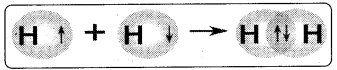A) s – s
B) p – p
C) s – p
D) None of these
A) s – s

Question 53.
The following diagram shows ……………….. overlap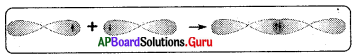A) s – s
B) p – p
C) s – p
D) None of these
B) p – pQuestion 54.
The number of σ and π bonds in Oxygen molecule is ………………..
A) 1,1
B) 1, 2
C) 1, 0
D) None of these
A) 1,1

Question 55.
The number of σ and π bonds in Nitrogen molecule is
A) 1, 0
B) 1, 2
C) 1,1
D) None of these
B) 1, 2

Question 56.
Match the following:
a) sp hybridisation — i) BF3
b) sp2 hybridisation — ii) BeCl2
c) sp3 hybridisation — iii) CH4, NH3, H2O
A) a → ii, b →i, c → iii
B) a → i, b → ii, c → iii
C) a → iii, b → ii, c → i
D) a →iii, b → i, c → ii
A) a → ii, b →i, c → iii

Question 57.
Which of the following statements are correct ?
A) sp3 hybridisation leads to tetrahy dral structure with bond angle 109° 28′
B) sp2 hybridisation leads to trigonal planar structure with bond angle 120°
C) sp hybridisation leads to linear structure with bond angle 180°
D) All of these
D) All of these

Question 58.
Ionic compounds are soluble in …………….
A) Benzene
B) Carbon tetra chloride
C) Water
D) Chloroform
C) Water

Question 59.
The overlap present in following diagram is……………..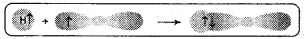A) s – s
B) s – p
C) p – p
D) None of these
B) s – p

Question 60.
Napthalene is soluble in …………….
A) Non – polar solvent
B) Kerosene
C) Water
D) Both A and B
D) Both A and B

Question 61.
The bond present in HCl is …………….
A) Ionic
B) Covalent
C) Polar covalent
D) Dative
A) Ionic

Question 62.
The following is not the characteristic of ionic compound
A) They are soluble in polar solvents like water
B) They have high melting and boiling points
C) Their solutions are bad conductors of electricity
D) They are crystalline solids
C) Their solutions are bad conductors of electricity

Question 63.
The number of hybrid orbitals formed in sp3 hybridisation is ……………
A) 1
B) 2
C) 3
D) 4
D) 4

Question 64.
Hybridisation is …………… of atomic orbitals.
A) exchange
B) interaction
C) intermixing
D) none of these
C) intermixing

Question 65.
The inert gas element which do not have octet configuration in the outermost orbit is ……………
A) Ar
B) Kr
C) Rn
D) He
D) HeCONCEPT – II: Electronic Theory of Valence: Ionic Bond

Question 66.
The ionic bond forms easily between which groups of elements ?
A) I-A and VII-A
B) II-A and V-A
C) I-A and V-A
D) II-A and VI-A
A) I-A and VII-A

Question 67.
Which of the following elements form a unipositive ion ?
A) Mg
B) Al
C) Ca
D) Na
D) Na

Question 68.
Which of the following anions has Ne electronic configuration?
A) Cl
B) O2-
C) P3-
D) Br
B) O2-

Question 69.
For the formation of ionic bond the electronegativity difference between atoms of two elements should be ………
A) greater than or equal to 1.9
B) less than or equal to 0.9
C) equal to 1.5
D) equal to 1
A) greater than or equal to 1.9

Question 70.
Which one of the following is not an ionic compound ?
a) BeCl2
b) BF3
c) NH3
d) CH4
A) a, b only
B) b, c only
C) a, b, c, d
D) None
C) a, b, c, d

Question 71.
Winch one of the following is wrong about NaCl crystal?
A) It does not conduct electricity in aqueous state
B) It is soluble in water
C) The coordination number of Cl in NaCl crystal is 6
D) It is face centered cubic crystal
A) It does not conduct electricity in aqueous state

Question 72.
The coordination number of Na+ and Cl in NaCl is ………….
A) 4
B) 6
C) 8
D) 2
B) 6

Question 73.
Generally the chemical bond formed between IA and VII A group elements …………….
A) Electrovalent bond
B) Covalent bond
C) Metallic bond
D) Dative bond
A) Electrovalent bond

Question 74.
The valency of aluminium in AI2O3 with respect to oxygen Is …….
A) 2
B) 3
C) 1
D) 1.5
B) 3Question 75.
The formula of the compound formed by A3+ and B2- is ……………..
A) A3 B2
B) A2 B3
C) AB
D) A2B
B) A2 B3

Question 76.
The ratio of coordination number of Na+ and Cl in face centred cubic lattice crystal of NaCl is …………
A) 6 : 6
B) 6 :1
C) 1 : 6
D) 3 : 4
A) 6 : 6

Question 77.
The coordination number of Na+ in NaCl is ……………….
A) 3
B) 4
C) 5
D) 6
D) 6

Question 78.
Which of the following element lose three electrons to get octet in its outer shell?
A) Cs
B) Mg
C) O
D) Al
D) Al

Question 79.
The element with negative charge having the electronic configuration 2,8,8 is ……………..
A) O2-
B) Co
C) Al3+
D) P2-
B) Co

Question 80.
The condition for the formation of ionic bond is ……………….
A) Elements involved should of equal size
B) High electropositivity of atom
C) Atoms should have low ionization potential
D) Elements with high electronegativity difference
D) Elements with high electronegativity difference

Question 81.
2, 8, 1 and 2, 8, 7 are electronic configurations of A and B elements combine to form a molecule. Its formula is ………….
A) AB
B) AB2
C) A2B
D) AB3
A) AB

Question 82.
Which of the following statements is not true ?
A) Valency of metal is its group number
B) Valency of non metal is equal to its group number
C) Noble gases are zero valent
D) Valency of non metal is 8 – group number
B) Valency of non metal is equal to its group numberQuestion 83.
The formula of metal (M) chloride is MCl3. Formula of its sulphate is
A) MS04
B) M2(S04)3
C) M(SO4)2
D) M(SO4)3
B) M2(S04)3

CONCEPT – III: Covalent Bond

Question 84.
Which of the following is a covalent compound ?
A) NaCl
B) NH3
C) MgCl2
D) LiF
B) NH3

Question 85.
The number of covalent bonds in methane is …………….
A) 1
B) 2
C) 3
D) 4
D) 4

Question 86.
The bond angle in BeF2 molecule is
A) 109° 28’
B) 120°
C) 180°
D) 104° 31′
C) 180°

Question 87.
The scientists proposed VSEPR theory are…………………
A) Sidgwick and Powell
B) Gillespie and Nyholm
C) Davy and Powell
D) Davy and Nyholm
A) Sidgwick and Powell

Question 88.
The molecule having 104°31′ as bond angle is ………………
A) CH4
B) H20
C) NH3
D) C2H4
B) H20

Question 89.
The molecule with two bond pairs in two covalent bonds around the nucleus of the central atom without any lone pair in the valance shell is
A) BeCl2
B) BF3
C) NH3
D) CH4
A) BeCl2

Question 90.
The molecules with decreasing order of
bond angles are
A) BF3, NH3, H2O, CH4
B) BeCl2, BF3, CH4, NH3
C) BCl3, H2O,NH3,CH4
D) BeCl2, H2O, NH3, CH4
B) BeCl2, BF3, CH4, NH3

Question 91.
The bond angle in Berylium chloride is……………..
A) 120°
B) 110°
C) 180°
D) 104.31°
C) 180°

Question 92.
The shape of H2O molecule is ……………..
A) Linear
B) V-Shape
C) Trigphal bipyramidal
D) Trigonal pyramidal
B) V-Shape

Question 93.
AH2 is formed when element A reacts with hydrogen. Then the number of electrons in valence shell of A are generally
A) 2
B) 3
C) 5
D) 8
A) 2Question 94.
The valence shell of the central atom of a molecule has four bond pairs of electrons. Shape of the molecule is ……………..
A) Linear
B) Plane triangular
C) Square planar
D) Tetrahydral
D) Tetrahydral

Question 95.
A molecule having 2 bond pairs and 2 lone pairs in the valence shell of its central atom.
A) H20
B) NH3
C) CH4
D) BF3
A) H20

CONCEPT – IV : VSEPRT

Question 96.
In a molecule AB3 there is a lone pair in the valence shell of central atom. The shape of molecule.
A) Tetrahydral
B) Linear
C) Pyramidal
D) Plane triangular
C) Pyramidal

Question 97.
The number of bond pairs and lone pair of electrons in O2 molecules respectively.
A) 2, 2
B) 2,1
C) 4, 2
D) 2, 4
D) 2, 4

Question 98.
Bond energy of HCl is
A) 410 KJ mol-1
B) 432 KJ mol-1
C) 460 KJ mol -1
D) 480 KJ mol-1
B) 432 KJ mol-1

CONCEPT – V : Valence Bond Theory – Hybridisation

Question 99.
A) C2H4
B) O2
C) N2
D) H2O
D) H2O

Question 100.
The type of hybridization in CH4 molecule is
A) sp
B) sp2
C) sp3
D) sp3d
C) sp3

Question 101.
Ionic compounds are soluble in
A) kerosene
B) benzene
C) water
D) ether
C) water

Question 102.
Which one of the following is not a polar molecule
A) H2O
B) HCl
C) NH3
D) BF3
D) BF3

Question 103.
Ionic compounds are soluble in
A) non – polar solvents
B) ether
C) polar solvents
D) CCl4
C) polar solvents

Question 104.
The number of σ bonds in CH4 molecule is …………….
A) 2
B) 3
C) 4
D) 1
C) 4

Question 105.
Which of the following molecule has highest bond dissociation energy.
A) F – F
B) Cl-Cl
C) Br – Br
D) I – I
B) Cl-Cl

Question 106.
The number of hybrid orbitals and atomic orbitals involved in BF3 respectively are ……………
A) 2, 2
B) 4, 4
C) 3/1
D) 3,3
D) 3,3

Question 107.
The molecule involves sp3 hybridiza¬tion is ……………..
A) CH4
B) NH3
C) H2O
D) All of these
D) All of these

Question 108.
Choose the correct form of the diagram representing H2 molecule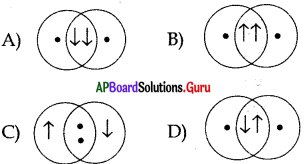D

Question 109.
The order of strength of overlapping is
A) s-s > p-p > s-p
B) s-p = s-s = p-p
C) s-p > s-s > p-p
D) p-p > s-p > s-s